# GSEB Solutions Class 10 Maths Chapter 6 Triangles Ex 6.6

Gujarat Board GSEB Solutions Class 10 Maths Chapter 6 Triangles Ex 6.6 Textbook Questions and Answers.

## Gujarat Board Textbook Solutions Class 10 Maths Chapter 6 Triangles Ex 6.6

Question 1.
In the figure, PS is the bisector of $$\angle QPR$$ of
$$\angle QPR$$. Prove that = $$\frac { QS }{ SR }$$ = $$\frac { PQ }{ PR }$$
Solution:
Given: ∆PQR in which PS is the bisector of $$\angle QPR$$.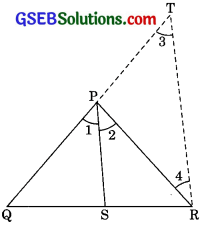To prove: $$\frac { QS }{ SR }$$ = $$\frac { PQ }{ PR }$$
Construction: Draw RT || PS, which meet QP produce at T.
Proof:
$$\angle 1$$ = $$\angle 2$$ …………. (1) (given)
PS || RT (by construction)
$$\angle 2$$ = $$\angle 4$$ …………. (2)
PR = PT
(side opposite to equal angles are equal)
In ∆QRT
$$\frac { QS }{ SR }$$ = $$\frac { PQ }{ PR }$$
$$\frac { QS }{ SR }$$ = $$\frac { PQ }{ PR }$$ (PT = PR)Question 2.
In the given figure, D is a point on hypotenuse AC of ∆ABC, such that BD ⊥ AC, DM ⊥BC and DN ⊥ AB. Prove that:
(i) DM2 = DN.
(ii) DM2 = DM . ANSolution:
In the given figure ∆ABC in which
AB ⊥ BC and DM ⊥ BC,
AB || DM
Similarly CB ⊥ AB and DN ⊥ AB
CB || DN
Hence quadrilateral BMDN is a rectangle
BM = ND
(i) In ∆BMD
$$\angle 1$$ + $$\angle BMD$$ + $$\angle 2$$ = 180°
(by Angle sum property)
$$\angle 1$$ + 90° +$$\angle 2$$ = 180°
$$\angle 1$$ + $$\angle 2$$ = 90° ……………(1)
Similarly, in ∆DMC we have
$$\angle 3$$ + $$\angle 4$$ = 90° ……………. (2)
Since BD ⊥ AC
Therefore $$\angle 2$$ + $$\angle 3$$ = 90° ………….(3)
From eqn (1) and (3)
$$\angle 1$$ + $$\angle 2$$ = $$\angle 2$$ + $$\angle 3$$
$$\angle 1$$ = $$\angle 3$$
Also from eqn (2) and (3)
$$\angle 3$$ + $$\angle 4$$ = $$\angle 2$$ + $$\angle 3$$
$$\angle 2$$ = $$\angle 4$$
Thus is ∆DMB and ∆DMC
We have
$$\angle 1$$ + $$\angle 3$$ and $$\angle 2$$ + $$\angle 4$$
∆BND ~ ∆DNA
$$\frac { BN }{ DN }$$ = $$\frac { ND }{ DA }$$
$$\frac { DM }{ DN }$$ = $$\frac { DN }{ MC }$$
DN2 = DM . MCQuestion 3.
In figure ABC is a triangle in which 90° and AD ⊥ CB produced prove that
AC2 = AB2 + BC2 + 2BC . BD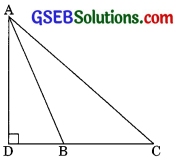Solution:
Given : ∆ABC in which < ABC > 90° and AD ⊥ CB produced.
To prove: In right triangle ADC
$$\angle D$$ = 90°
AC2 = AD2 + DC2
(by Pythagoras theorem)
AC2 = AD2 + (BD + BC)2
(DC = BD + BC)
AC2 = AD2 + BD2 + BC2 + 2BC . BD ………….(1)
Now in right ∆ADB
AC2 = AD2 + BD2 ………………….(2)
(by Pythagoras theorem)
From eqn (1) and (2)
AC2 = AB2 + BC2 + 2BC . BDQuestion 4.
In figure, ABC is a triangle in which $$\angle ABC$$ $$\angle 90°$$ and AD ⊥ BC.
To Prove: AC2 = AB2 + BC2 + 2BC . BD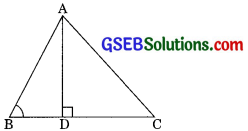Proof: In right ∆ADC
$$\angle D$$ = 90°
AC2 = AD2 + (BC – BD)2
(DC = BC – BD)
AC2 = AD2 + BC2 + BD2 – 2BC . BD
AC2 = AD2 + BD2 + BC2 – 2BC . BD ……………(1)
Now in right ∆ADB
AB2 = AD2 + BD2 …………(2)
(by Pythagoras theorem)
From eqn (1) and (2) we have
AC2 = AB2 + BC2 – 2BC2 . BDQuestion 5.
In figure AD is a median of a triangle ABC and AM ⊥BC prove that
(i) AC2 = AD2 + BC . DM + $$\left( \frac { BC }{ 2 } \right)$$2
(ii) AB2 = AD2 – BC . DM + $$\left( \frac { BC }{ 2 } \right)$$2
(iii) AC2 + AB2 = 2AD2 + $$\frac { 1 }{ 2 }$$BC2Solution:
AD is the mediam of ∆ABC and AM ⊥ BC.
To prove:
(i) AC2 = AD2 + BC . DM + $$\left( \frac { BC }{ 2 } \right)$$2
(ii) AB2 = AD2 – BC . DM + $$\left( \frac { BC }{ 2 } \right)$$2
(iii) AC2 + AB2 = 2AD2 + $$\frac { 1 }{ 2 }$$BC2
Proof:
(i) In right ∆AMC
$$\angle M$$ = 90°
AC2 = AM2 + MC2
(by Pythagoras theorem)
since MC = DM + DC we have,
AC2 = AM2 + (DM + DC)2
AC2 = AM2 + DM2 + DC2 + 2DM . DC $$\left( DC=\frac { BC }{ 2 } \right)$$
AC2 = AM2 + DM2 + DM . BC + $$\left( \frac { BC }{ 2 } \right)$$2 + 2MD . $$\frac { BC }{ 2 }$$
AC2 = AM2 + DM2 + DM . BC + $$\left( \frac { BC }{ 2 } \right)$$2 ……………(1)
Now in ∆AMD
AD2 = AM2 + DM2 ……………….(2)
(by Pythagoras theorem)
From eqn (1) and (2) we have
AC2 = AD2 + BC . DM + $$\left( \frac { BC }{ 2 } \right)$$2 …………(3)

(ii) In right ∆AMB
AB2 = AM2 + BM2
(by Pythagoras theorem)
AB2 = AM2 + (BD – DM)2
(BM = BD – DM)
AB2 = AM2 + BD2 – 2BD . DM + BD2
AB2 = AM2 + DM2 – $$\frac { 2BC.DM }{ 2 }$$ + $$\left( \frac { BC }{ 2 } \right)$$2 $$\left( BD=\frac { BC }{ 2 } \right)$$ ………..(4)
From eqn (2) and (4)
AB2 = AD2 – BC2 . DM + $$\left( \frac { BC }{ 2 } \right)$$2 …………….(5)

(iii) Adding eqn (3) and (5) we have
AC2 + AB2 = 2AD2 + 2$$\left( \frac { BC }{ 2 } \right)$$2
AC2 + AB2 = 2AD2 + 2 . $$\left( \frac { { BC }^{ 2 } }{ 4 } \right)$$
AC2 + AB2 = 2AD2 + 2 . $$\left( \frac { { BC }^{ 2 } }{ 2 } \right)$$Question 6.
Prove that the sum of the squares of the diagonals of parallelogram is equal to the sum of the squares of its sides.
Solution:
Given:
ABCD is a ||gm whose diagonals are AC and BD.To prove:
AB2 + BC2 + CD2 + CA2 = AC2 + BD2
Construction:
Draw AM ⊥ DC and BN ⊥ DC (produced)
Proof: In right ∆AMD and ∆BNC
AD = BC (opposite sides of ||gm)
AM = BN (altitudes of same ||gm on the same base)
∆AMD $$\cong$$ ∆BNC (by RHS congruence Rule)
MD = CN …………..(1) (by CPCT)
Now in right triangle BND
$$\angle N$$ = 90°
BD2 = BN2 + DN2
(by Pythagoras theorem)
BD2 = BN2 + (DC + CN)2(DN = DC + CN)
BD2 = BN2 + DC2 CN2 + 2DC . CN
BD2 = BN2 + CN2 + DC2 + 2DC . CN ………….(2)
Now in right ∆BNC
BD2 = BN2 + CN2 ……..(3)
From eqn (2) and (3)
BD2 = BC2 + DC2 + 2DC . CN ………………(4)
Now in right ∆ADM
$$\angle M$$ = 90°
AC2 = AM2 + MC2 ………….
(by Pythagoras theorem)
AC2 = AM2 + (DC – DM)2
(MC = DC – DM)
AC2 = AM2 + DC2 + DM2 – 2DC . DM
AC2 = AM2 + DM2 + DC2 – 2DC . DM …………..(5)
Now in right ∆ADM
AD2 = AM2 + DM2 ……………(6)
(by Pythagors theorem)
From eqn (5) and (6) and we have
AC2 = AD2 + DC2 – 2DC . DM
AC2 = AD2 + AB2 – 2DC . CN …………..(7)
(DC = AB opposite sides of ||gm and DM = CN from eqn (1))
Adding eqn (4) and (7)
AC2 + BD2 = AD2 + AB2 + BC2 + DC2
AC2 + BD2 = AB2 + BC2 + CD2 + DA2Question 7.
In figure, two chords AB and CD intersect each other at the point P prove that
(i) ∆APC ~ ∆DPB
(ii) AP . PB = CP . DP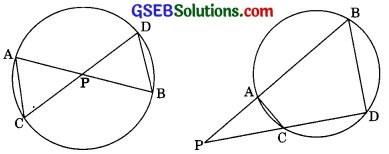Solution:
Given: Two chords AB and CD intersects each other at the points P.
To Prove: (i) ∆APC ~ ∆DPB
(ii) AP . PB = CP . DP
Proof:
(i) ∆APC and ∆DPB
$$\angle APC$$ = $$\angle DPB$$
(Vertically opposite angles)
$$\angle CAP$$ = $$\angle BDP$$
(Angles in the same segment)
∆APC ~ ∆DPB
(by AA similarity)

(ii) $$\frac { AP }{ DP }$$ = $$\frac { CP }{ PB }$$ (∆APC ~ ∆DPB) Prove above.
(Corresponding sides of two similar triangle are proportional)
AP . PR = CP . DPQuestion 8.
In figure, two chords AB and CD of a circle intersect each other at the point P (When produce) outside the circle. Prove that
(i) ∆PAC ~ ∆PDB
(ii) PA . PB = PC . PDSolution :
Given: AB and CD are two chords of a circle intersect each othe rat the point P when produced outside the circle.
To prove:
(i) ∆PAC ~ ∆PDB
(ii) PA . PB = PC . PD
Proof:
In ∆PAC and ∆PDB
(In a cyclic quadrilateral the exterior angle is equal to the interior opposite angle)
Here,
$$\angle PAC$$ = $$\angle PDB$$ …………………(1)
$$\angle PCA$$ = $$\angle PBD$$ …………………(2)
From eqn (1) and (2)
In ∆PAC and ∆PDB
(by AA similarity)

(iii) We have, ∆PAC ~ ∆PDB
(proved above)
$$\frac { PA }{ PD }$$ = $$\frac { PC }{ PB }$$
(corresponding sides of the similar triangles are proportional)
PA . PB = PC . PDQuestion 9.
In figure, D is a point on side BC of ∆ABC such that $$\frac { BD }{ CD }$$ = $$\frac { AB }{ AC }$$.
Prove that AD is the bisector of $$\angle BAC$$.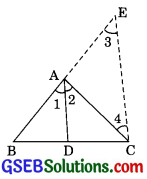Solution:
Given: D is the point on side BC of ∆ABC such that $$\frac { BD }{ CD }$$ = $$\frac { AB }{ AC }$$
To prove: Ad is a bisector of $$\angle BAC$$ i.e.
$$\angle BAD$$ = $$\angle CAD$$
Construction: Produced BA to E such that
AE = AC. Join CE
Proof: We have $$\frac { BD }{ CD }$$ = $$\frac { AB }{ AC }$$
$$\frac { BD }{ CD }$$ = $$\frac { AB }{ AE }$$ (AC = AE by construction)
AD || CE (by converse of BPT) ……………..(1)
$$\angle 1$$ = $$\angle 3$$
(Corresponding angle) …………..(2)
$$\angle 2$$ = $$\angle 4$$
(Alternate Interior angles) ……..(3)
$$\angle 3$$ = $$\angle 4$$
(Angle opposite to equal sides are equal)
From eqn (1), (2) and (3)
$$\angle 1$$ = $$\angle 2$$Question 10.
Nazima is fly fishing in a stream. The tip of her fishing rod is 1.8 m above the surface of the water and the fly at the end of the string rests on the water 3.6 m away and 2.4 m from a point directly under the tip of the rod. Assuming that her string (from the tip of her rod to the fly) is taut, how much string does she have out (see figure)? If she pulls in the string at the rate of 5 cm per second, what will be the horizontal distance of the fly from her after 12 seconds?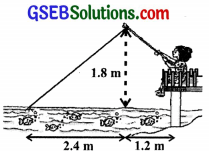Solution:
Let us find the length of the string that the has out
In right ∆ABC
AC2 = AB2 + BC2
(by Pythagoras theorem)
AC2 = (2.4)2 + (1.8)2
AC2 = 5.76 + 3.24 = 9
AC = 3m
i.e length of string she has out = 3m since the string is pulled out at the rate of 5 cm/sec.
Length of the string pulled out in
12 seconds = 5 cm x 12 cm = 60 cm
= $$\frac { 60 }{ 100 }$$ m = 0.60 metre
Remaining string left out = (3 – 0.60) m
= 2.4m
To find horizontal distance
Inright ∆PBC, let PB the required horizontal distance of fly.
Since PC2 = PB2 + BC2
PB2 = PC2 + BC2
PB2 = (2.4)2 – (1.8)2
PB2 = 5.76 – 3.24
PB2 = 2.52
PB = 1.59 (approx)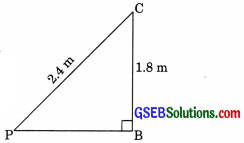Thus, the horizontal distance of the fly from nazima after 12 seconds
= 1.59 + 1.2 = 2.79 m (approx)